# Civil Engineering - Steel Structure Design

### Exercise :: Steel Structure Design - Section 2

6.

An axial loaded column is supported on a slab base whose projection is 8 cm. If the intensity of pressure from concrete is 40 kg/cm2 and allowable bending stress in slab base is 1890 kg/cm2, the thickness (t) of the slab base, is

 A.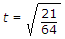B.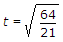C.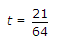D.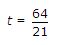Explanation:

No answer description available for this question. Let us discuss.

7.

If P is the load applied to a bracket with an eccentricity e, the resisting moment F exerted by a rivet at a distance rn from the centre of gravity, is

 A.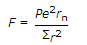B.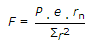C.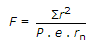D.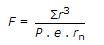E.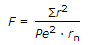Explanation:

No answer description available for this question. Let us discuss.

8.

The effective length of a weld, is taken as the actual length

 A. minus the size of weld B. minus twice the size of weld C. plus the size of weld D. plus twice the size of weld E. none of these.

Explanation:

No answer description available for this question. Let us discuss.

9.

The permissible bearing stress in steel, is

 A. 1500 kg/cm2 B. 1890 kg/cm2 C. 2025 kg/cm2 D. 2340 kg/cm2 E. 2250 kg/cm2

Explanation:

No answer description available for this question. Let us discuss.

10.

The main assumption of the method of simple design of steel frame work, is :

 A. beams are simply supported B. all connections of beams, girders and trusses are virtually flexible C. members in compression are subjected to forces applied at appropriate eccentricities D. all the above.